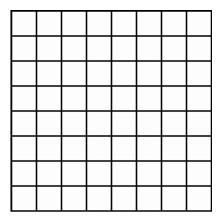Stereographic Projection from Four-Space

We have described features of stereographic projection from the sphere in three-space to a plane. To describe this technique in the next higher dimension, we consider the effect of central projection on the analogue of a sphere in four-dimensional space, which we call a hypersphere. The ordinary sphere in three-space is the collection of points at a fixed distance from a given point. In four-space, a hypersphere is defined the same way, as the collection of all points at fixed distance from a given point. All vertices of a cube are at the same distance from its center, so the vertices of a cube are situated on a sphere about its center. Similarly, all vertices of a hypercube are situated on a hypersphere.Eight-by-eight subdivision of the plan for the polyhedral torus.

All of the vertices of the four-by-four polyhedral torus studied earlier in this chapter lie on a hypersphere since the vertices of this torus are the 16 vertices of the hypercube. In a similar way, we can assemble an eight-by-eight grid of 64 small squares in four-space so that all of its vertices lie on a hypersphere. We may use central projection to find the image of this polyhedral torus in three-space, and then look at its projections on the computer screen to see pictures of polyhedra in space which better approximate the smooth torus of revolution. Subdividing again and again produces a very close approximation of a surface in four-space having the smooth torus as its image under central projection. This particular torus, first studied in the last century by William Clifford, is known as the Clifford torus. This surface in four-space is of extreme importance in geometry and topology because of its remarkable symmetries, and it appears as well in the physics of dynamical systems, for example as a surface defined by equations governing the position and velocity of a pair of pendulums.

Central projection of the Clifford torus into three-space gives the familiar torus of revolution. As we saw in Chapter 3, the torus of revolution is covered by four different families of circles: the horizontal circles of latitude, the vertical circles of longitude, and two types of circles in planes making a 45-degree angle with the horizontal. Since central projection preserves circles on a hypersphere, the Clifford torus must also be covered by four families of circles. If we rotate the hypersphere containing the torus, keeping the light source fixed, then the images of the Clifford torus under stereographic projection become distorted, forming a collection of objects called cyclides of Dupin after their discoverer, Claude Dupin.

We can display the structure of the Clifford torus in four-space by dividing the surface into cylindrical bands and removing half of them. As we rotate the hypersphere in four-space, some portions of the torus have more accurate images and other parts become distorted. If we rotate so that one of the points of the torus goes through the Iight source, then the image in three-dimensional space stretches out to infinity. This infinitely large surface is remarkable in that, like the plane, it totally and symmetrically separates all of space into two congruent pieces. As we continue the rotation in four-dimensional space, the image returns to a familiar torus of revolution but with an essential difference: the cylindrical bands that formerly were parallels of latitude have now become meridians of longitude. During this deformation, the torus has turned completely inside out! Latitude and longitude have interchanged.

In the next chapter we will use this central projection of a torus on the hypersphere to model the motion of a pair of pendulums.

Here is a movie of the torus rotating in three-space and in four-space.